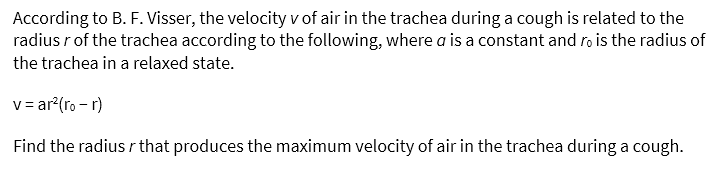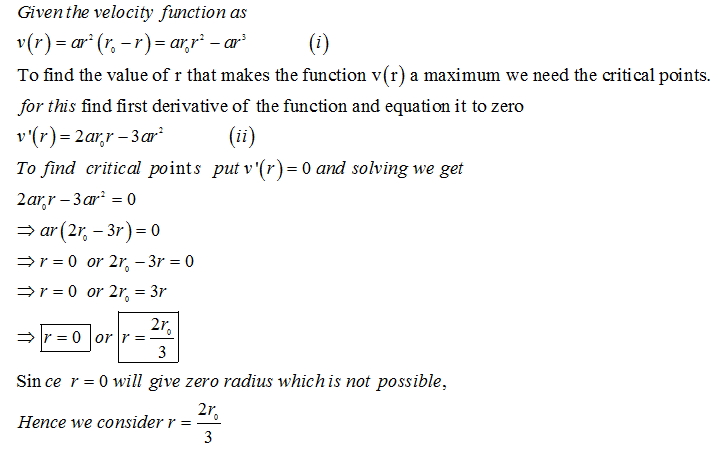# According to B. F. Visser, the velocity v of air in the trachea during a cough is related to the radius r of the trachea according to the following, where a is a constant and r0 is the radius of the trachea in a relaxed state.v = ar2(r0 − r)Find the radius r that produces the maximum velocity of air in the trachea during a cough.

Question
2 views

According to B. F. Visser, the velocity v of air in the trachea during a cough is related to the radius r of the trachea according to the following, where a is a constant and r0 is the radius of the trachea in a relaxed state.

v = ar2(r0 − r)

Find the radius r that produces the maximum velocity of air in the trachea during a cough.

check_circle

Step 1Step 2...

### Want to see the full answer?

See Solution

#### Want to see this answer and more?

Solutions are written by subject experts who are available 24/7. Questions are typically answered within 1 hour.*

See Solution
*Response times may vary by subject and question.
Tagged in

### Calculus